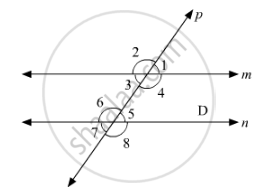# In the Below Fig, Transversal 𝑙 Intersects Two Lines M and N, ∠4 = 110° and ∠7 = 65°. is M || N? - Mathematics

In the below fig, transversal 𝑙 intersects two lines m and n, ∠4 = 110° and ∠7 = 65°. Is m || n?#### Solution 1

Given:

∠4 = 110°, ∠7 = 65°

To find: Is m || n

Here, ∠7 =∠5= 65°       [Vertically opposite angle]

Now,

∠4 + ∠5 = 110 + 65° = 175°

∴m is not parallel to n as the pair of co-interior angles is not supplementary.

#### Solution 2

The figure is given as follows:It is given that l is a transversal to lines m and n. Also,

∠4 = 110° and ∠7 = 65°.

We need check whether m||n or not.

We have.∠7 = 65°.

Also,∠7 5.and  ∠5are vertically opposite angles, thus, these two must be equal. That is,

∠5 = °65.

Also, ∠4 =110°

Adding this equation to (i), we get:

∠4 + ∠5 =110° +65°

∠4 +∠5 =175°

But these are the consecutive interior angles which are not supplementary.

Theorem states: If a transversal intersects two lines in such a way that a pair of consecutive interior angles is supplementary, then the two lines are parallel.

Thus, m is not parallel to n.

Concept: Pairs of Angles
Is there an error in this question or solution?
Chapter 10: Lines and Angles - Exercise 10.4 [Page 47]

#### APPEARS IN

RD Sharma Mathematics for Class 9
Chapter 10 Lines and Angles
Exercise 10.4 | Q 15 | Page 47

Share